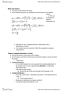ECO220Y1 Lecture 14: Lecture 14

23 views4 pages
School
Department
Course
Professorvioletprairie-dog339 and 90 others unlocked27
Verified Note
27 documents

Document Summary

Lecture 14 testing hypotheses about proportions ii. P-value: probability of observing something as extreme as our sample if the null was true. If p-value is small enough , we can reject the null hypothesis: this lecture: Relationship between con dence intervals and hypothesis testing. Common ones: 0. 1, 0. 05 or 0. 01. Very often: 0. 05: if the p-value is smaller than , we reject h0, if the p-value is greater than or equal to , we fail to reject h0. H0: how to phrase the conclusion: we reject/fail to reject the null hypothesis at the signi cance level. , notice that it may still be useful to report p-values, since they are more informative. We would fail to reject the null hypothesis if p-value 0. 101, but reject if p-value 0. 099. 3 di erence between statistical and economic signi cance: statistical signi cance: statistically di erent from the hypothesized value of the parameter. 4 critical values: the values are probabilities.

Get access

\$8 USD/m\$10 USD/m
Billed \$96 USD annuallyHomework Help
Study Guides
Textbook Solutions
Class Notes
Textbook Notes
Booster Class
Class+
\$8 USD/m
Billed \$96 USD annuallyHomework Help
Study Guides
Textbook Solutions
Class Notes
Textbook Notes
Booster Class